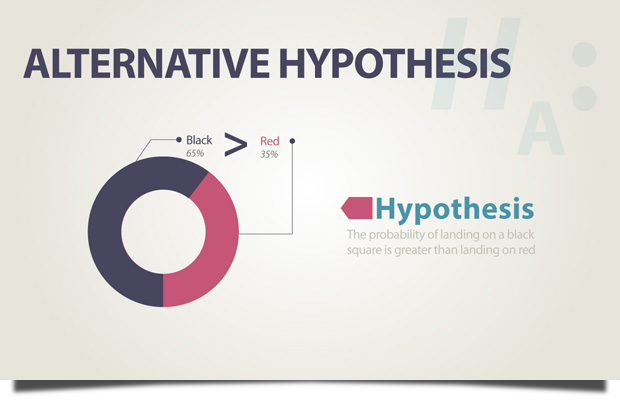# Alternative Hypothesis

Alternative Hypothesis is a statement about the parameters that we believe to be true if the null hypothesis is not true.

- Triola (2009)

# ExampleIn the null hypothesis example it states that the probability of landing on the black square has the same probability of landing on the red square. Then the alternative hypothesis states that the probability of landing on a black square is greater than landing on red.

Hypothesis

Null Hypothesis